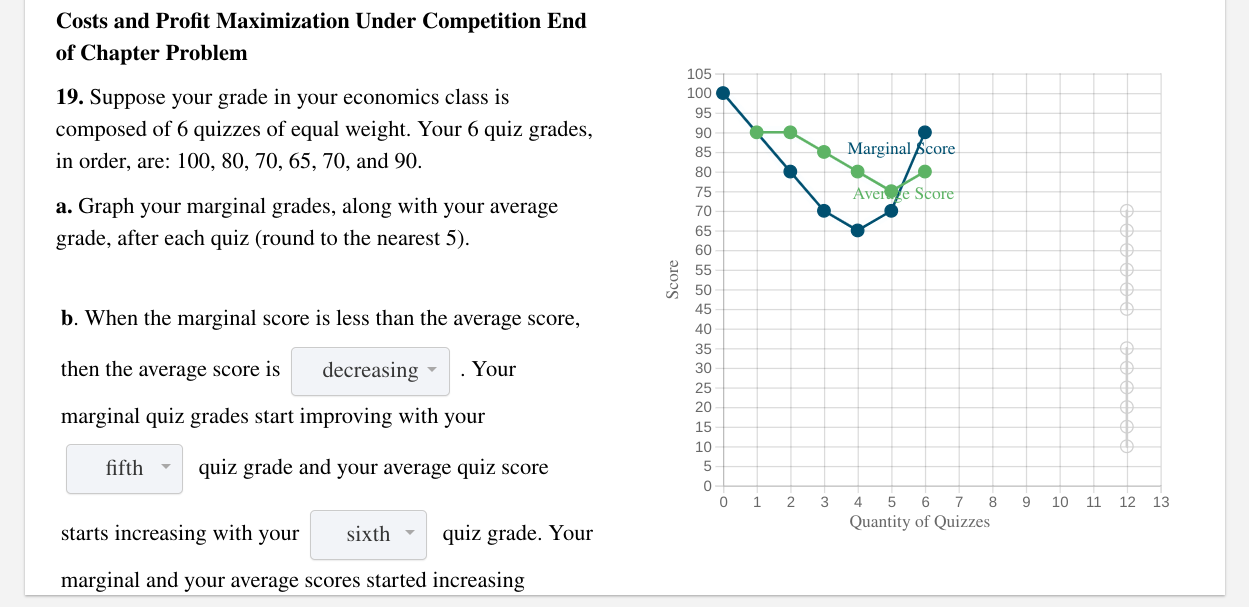# (Solved): Costs And Profit Maximization Under Competition End Of Chapter Problem 105 100 19. Suppose Your Grad...The graph for 19) a

Costs and Profit Maximization Under Competition End of Chapter Problem 105 100 19. Suppose your grade in your economics class is composed of 6 quizzes of equal weight. Your 6 quiz grades, in order, are: 100, 80, 70, 65, 70, and 90. Marginal Score Avere Score a. Graph your marginal grades, along with your average grade, after each quiz (round to the nearest 5). Score b. When the marginal score is less than the average score, then the average score is decreasing . Your marginal quiz grades start improving with your fifth - quiz grade and your average quiz score 0 1 2 3 9 10 11 12 13 4 5 6 7 8 Quantity of Quizzes starts increasing with your sixth - quiz grade. Your marginal and your average scores started increasing

We have an Answer from Expert